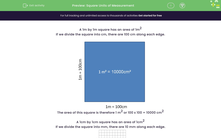# Square Units of Measurement

In this worksheet, students convert between mm2, cm2 and mm2.Key stage:  KS 3

Curriculum topic:   Number

Curriculum subtopic:   Use Standard Units of Measure

Difficulty level:#### Worksheet Overview

In this activity, we are  going to be finding the area of shapes, converting into different units where necessary.

To find the area of a square, we multiply the length x the width.

A 1 m by 1 m square has an area of 1 m2

If we divide the square into cm, there are 100 cm along each edge.The area of this square is therefore 1 m x 1 m = 1 m2 or 100 cm x 100 cm = 10,000 cm2

A 1 cm by 1 cm square has an area of 1 cm

If we divide the square into mm, there are 10 mm along each edge.The area of this square is therefore 1 cm x 1 cm = 1 cm2 or 10 mm  x 10 mm = 100 mm2

Have you got that?

Be very careful if you have to convert between measurements, when finding areas or volumes - make sure that you convert to the correct unit before you do the calculation. If you convert after you've done the calculation, you will probably get the wrong answer!### What is EdPlace?

We're your National Curriculum aligned online education content provider helping each child succeed in English, maths and science from year 1 to GCSE. With an EdPlace account you’ll be able to track and measure progress, helping each child achieve their best. We build confidence and attainment by personalising each child’s learning at a level that suits them.

Get started